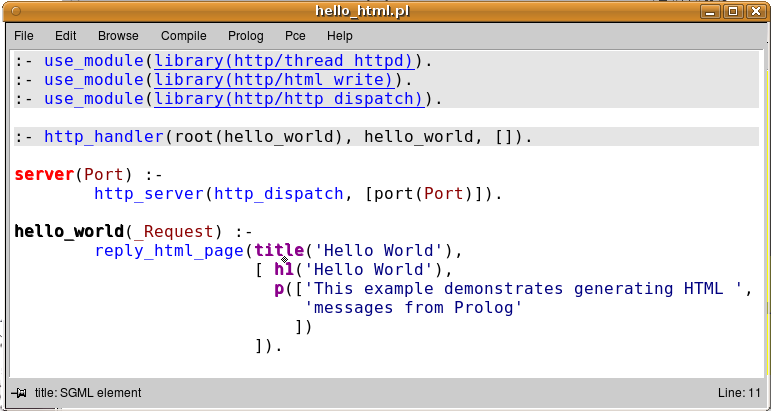Did you know ... Search Documentation:Hello World in HTML

HTML can be written the same way as in Hello World, using the `Content-type: text/html` and using format/2 to emit HTML tags.

However, this is a rather error-prone process. This page introduces library(http/html_write), which translates Prolog terms into well-formed HTML. We start with the simple example below.

```:- use_module(library(http/thread_httpd)).
:- use_module(library(http/http_dispatch)).
:- use_module(library(http/html_write)).		% new

:- http_handler(root(hello_world), say_hi, []).

server(Port) :-
http_server(http_dispatch, [port(Port)]).

say_hi(_Request) :-
[ h1('Hello World'),
p(['This example demonstrates generating HTML ',
'messages from Prolog'
])
]).```

The predicate reply_html_page/2 takes a description of the head and body of the HTML page and passes these to html//1. The argument to html//1 is either a list of terms or a single term. The functor-name of each term is an HTML-_tag_. It takes one or two arguments. If there is one argument, it is the HTML-_content_, again a term or a list of terms. If here are two arguments, the first specifies the HTML-_attributes_ and the second the content. Again, the attributes is a term or a list of terms, but these terms are of the form Attribute(Value) or Attribute=Value, at your choice.

There is one exception to this rule: if an HTML-_tag_ cannot have a content (e.g., <img>), the first argument are the attributes.

Below are a some examples. (1) shows specifying an attribute; (2) shows that <img> has only one argument that is interpreted as an attributes (when in doubt, using `img(<attributes>, [])` also works); (3) show lists of terms, (4 and 5) show that & and <> should not be escaped.

```1.	span(class(product_class), 'Computers'),

2.	img([width(32),height(32),src('/icons/computer.png')]),

3.	table([ tr([ td('cell 1a'), td('cell 1b')]),
tr([ td('cell 2a'), td('cell 2b')])
]),

4.	td(class(authors), 'Clocksin & Mellish'),

5.	p(['we can also use ', i('A<B'), ' without escaping.'])```

Now, you may ask "Where's the beef?" True, this syntax is surely less readable than HTML or XML. This way of producing web-pages is intended for dynamic web-pages. Fully static pages are much better served from files. For mixed files with large static parts and e.g., a dynamic table, PWP is the Prolog-based answer to PHP, ASP, JSP, etc.

One way to deploy the above described library(http/html_write) is to use Prolog data-manipulation to create the Prolog term that describes the body and then pass it to reply_html_page/2. The other is to use the rule definition facilities.

Note that PceEmacs has support for colouring HTML elements: